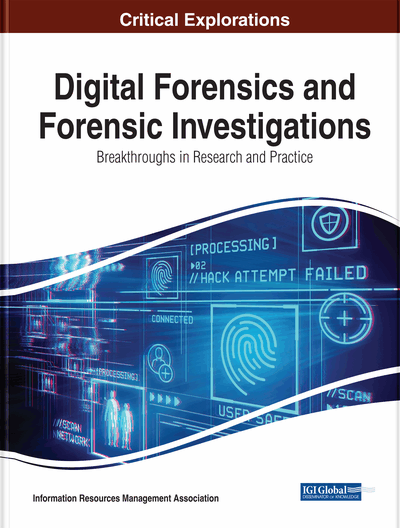# Contrast Modification Forensics Algorithm Based on Merged Weight Histogram of Run Length

Liang Yang (Nankai University, Tianjin, China), Tiegang Gao (College of Software, Nankai University, Tianjin, China), Yan Xuan (Nankai University, Tianjin, China) and Hang Gao (Nankai University, Tianjin, China)
DOI: 10.4018/978-1-7998-3025-2.ch031
Available
\$37.50
No Current Special Offers

## Abstract

A novel image forensic algorithm against contrast modification based on merged weight histogram of run length is proposed. In the proposed algorithm, the run length histogram features were firstly extracted, and then those of different orientation were subsequently merged; after normalization of the prior features, the authors calculated leaps in the histogram numerically; lastly, the generated features of authentic and tampered images were trained by a SVM classifier. Large amounts of experiments show that, the proposed algorithm has low cost of computation complexity, compared with some existing scheme, and it has better performance with many test databases, furthermore, the proposed algorithm can effectively detect local contrast modification of image.
Chapter Preview
Top

## Preliminaries

### Contrast Enhancement Detection Based on Histogram

Stamm et al. (2010) have proposed an algorithm of contrast enhancement detection based on histogram peak/gap artifacts left by attacked image; the main steps are described in the following:

• 1.

Calculate the image’s pixel value histogram h(x) and the modified histogram g(x) such that:g(x) = p(x)h(x)(1)

where, p(x) is a pinch off function, whose role is to eliminate the low end or high end saturated effect in image.where, N is the total number of pixels, G(k) is the discrete Fourier frequency transform of g(x), and β(k) is the cutoff function deemphasizing the low frequency components of G(k):(3) where, T corresponds to a desired cutoff frequency.

• 3.

At last, for a given threshold τ, F is compared with the τ to determined whether the image has been modified.

## Complete Chapter List

Search this Book:
Reset